# The Ishigami function¶

The Ishigami function of Ishigami & Homma (1990) is recurrent test case for sensitivity analysis methods and uncertainty.

Let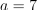and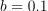(see Crestaux et al. (2007) and Marrel et al. (2009)). We consider the function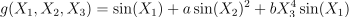for any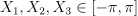We assume that the random variables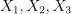are independent and have the uniform marginal distribution in the interval from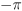to: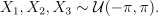## Analysis¶

The expectation and the variance of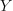are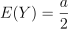and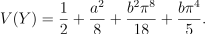The Sobol’ decomposition variances are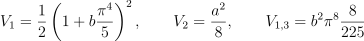and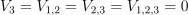.

This leads to the following first order Sobol’ indices: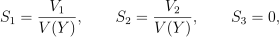and the following total order indices: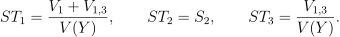The third variable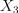has no effect at first order (because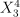it is multiplied by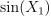), but has a total effet because of the interactions with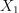. On the other hand, the second variable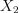has no interactions which implies that the first order indice is equal to the total order indice for this input variable.

We can load this model from the use cases module as follows :

>>> from openturns.usecases import ishigami_function
>>> # Load the Ishigami use case
>>> im = ishigami_function.IshigamiModel()


## API documentation¶

class IshigamiModel

Data class for the Ishigami model.

Examples

>>> from openturns.usecases import ishigami_function
>>> # Load the Ishigami model
>>> im = ishigami_function.IshigamiModel()

Attributes:
dimThe dimension of the problem

dim = 3

aConstant

a = 7.0

bConstant

b = 0.1

X1Uniform distribution

First marginal, ot.Uniform(-np.pi, np.pi)

X2Uniform distribution

Second marginal, ot.Uniform(-np.pi, np.pi)

X3Uniform distribution

Third marginal, ot.Uniform(-np.pi, np.pi)

distributionXComposedDistribution

The joint distribution of the input parameters.

ishigamiSymbolicFunction

The Ishigami model with a, b as variables.

modelParametricFunction

The Ishigami model with the a=7.0 and b=0.1 parameters fixed.

expectationConstant

Expectation of the output variable.

varianceConstant

Variance of the output variable.

S1Constant

First order Sobol index number 1

S2Constant

First order Sobol index number 2

S3Constant

First order Sobol index number 3

S12Constant

Second order Sobol index for marginals 1 and 2.

S13Constant

Second order Sobol index for marginals 1 and 3.

S23Constant

Second order Sobol index for marginals 2 and 3.

S123Constant
ST1Constant

Total order Sobol index number 1.

ST2Constant

Total order Sobol index number 2.

ST3Constant

Total order Sobol index number 3.

## Examples based on this use case¶Estimate correlation coefficients

Estimate correlation coefficientsVisualize sensitivity

Visualize sensitivityCompute grouped indices for the Ishigami function

Compute grouped indices for the Ishigami functionValidate a polynomial chaos

Validate a polynomial chaosCreate a polynomial chaos for the Ishigami function: a quick start guide to polynomial chaos

Create a polynomial chaos for the Ishigami function: a quick start guide to polynomial chaosChaos cross-validation

Chaos cross-validationCreate a sparse chaos by integration

Create a sparse chaos by integrationKriging: propagate uncertainties

Kriging: propagate uncertaintiesEvaluate the mean of a random vector by simulations

Evaluate the mean of a random vector by simulationsFAST sensitivity indices

FAST sensitivity indicesEstimate Sobol’ indices for the Ishigami function by a sampling method: a quick start guide to sensitivity analysis

Estimate Sobol' indices for the Ishigami function by a sampling method: a quick start guide to sensitivity analysisThe HSIC sensitivity indices: the Ishigami model

The HSIC sensitivity indices: the Ishigami model